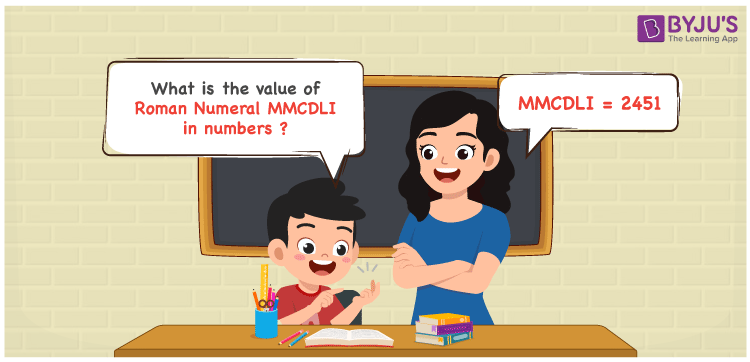Checkout JEE MAINS 2022 Question Paper Analysis : Checkout JEE MAINS 2022 Question Paper Analysis :

# MMCDLI Roman Numerals

MMCDLI Roman Numerals is 2451. In this article, MMCDLI is expanded and the number for each English alphabet is mentioned to get the numbers. The fundamental Maths operation addition has to be learned well in order to get the numeric form of MMCDLI. Roman numerals articles contain all the information needed to perform well in the annual exams. Therefore, MMCDLI can be indicated as 2451 in numbers.

 Number Roman Numeral 2451 MMCDLI## How to Write MMCDLI Roman Numerals in Numbers?

MMCDLI is expanded and converted to numbers in this section to provide a fun learning experience for the students.

MMCDLI = M + M + (D – C) + L + I

MMCDLI = 1000 + 1000 + (500 – 100) + 50 + 1

MMCDLI = 2451

## Video Lesson on Roman Numerals## Frequently Asked Questions on MMCDLI Roman Numerals

### Why is the roman numeral of 2451 written as MMCDLI?

We know that

MMCDLI = M + M + (D – C) + L + I

MMCDLI = 1000 + 1000 + (500 – 100) + 50 + 1

MMCDLI = 2451

### Determine the value of 2500 – 49.

We know that

2500 – 49 = 2451

Hence, the value of 2500 – 49 is 2451 which is written as MMCDLI.

### What is the remainder we obtain when MMCDLI is divided by XIII?

We know that

MMCDLI = 2451

XIII = 13

So the remainder we obtain when MMCDLI is divided by XIII is VII.